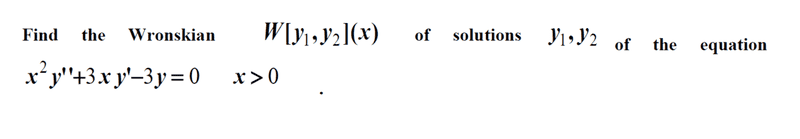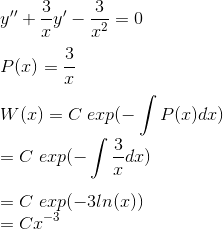# Find the Wronskian of solutions y1 and y2 of the equation

Fatima Hasan

## Homework Statement

[/B]## Homework Equations

##W(x) = C exp (∫ P(x) dx)##
##y''+P(x)y'+g(x)=0##

## The Attempt at a Solution

Divide the origin equation by (x) to get the standard form of the homogeneous equation :
##y'' +\frac{3y'}{x} - \frac{3y}{x}##
##P(x) = \frac{3}{x}##
## exp (- ∫ \frac{3}{x}##
## = exp ( -3 ln (x))##
## = x^{-3}##
##W(x) = C x^{-3} ##

Mentor

## Homework Statement

[/B]
View attachment 234516

## Homework Equations

##W(x) = C exp (∫ P(x) dx)##
##y''+P(x)y'+g(x)=0##

## The Attempt at a Solution

Divide the origin equation by (x)
No, you need to divide by ##x^2##
Fatima Hasan said:
to get the standard form of the homogeneous equation :
##y'' +\frac{3y'}{x} - \frac{3y}{x}##
The third term is incorrect. Also, you lost the = so you no longer have an equation.
Fatima Hasan said:
##P(x) = \frac{3}{x}##
## exp (- ∫ \frac{3}{x}##
## = exp ( -3 ln (x))##
## = x^{-3}##
##W(x) = C x^{-3} ##

Fatima Hasan
No, you need to divide by ##x^2##
The third term is incorrect. Also, you lost the = so you no longer have an equation.
I've solved it again , here's my work :#### Attachments

Mentor
Looks fine to me.

•Fatima Hasan